# SAT Math Multiple Choice Question 23: Answer and Explanation

### Test Information

Question: 23

3. If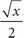= 2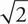, what is the value of x ?

• A. 4
• B. 16
• C. 16• D. 32

D Plug in the answers, starting with (B). If x = 16, the left side of the equation is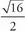=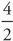= 2. Does that equal 2? No-it's too small. Choice (C) is ugly to work with, so try (D) next. If it is too big, (C) is your answer. For (D), x = 32, and the left side of the equation becomes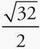=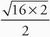=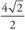= 2. It's a match, so (D) is correct. You could also solve this algebraically. Multiply both sides by 2 to get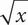= 4. Square both sides to get x = 16 × 2 = 32.